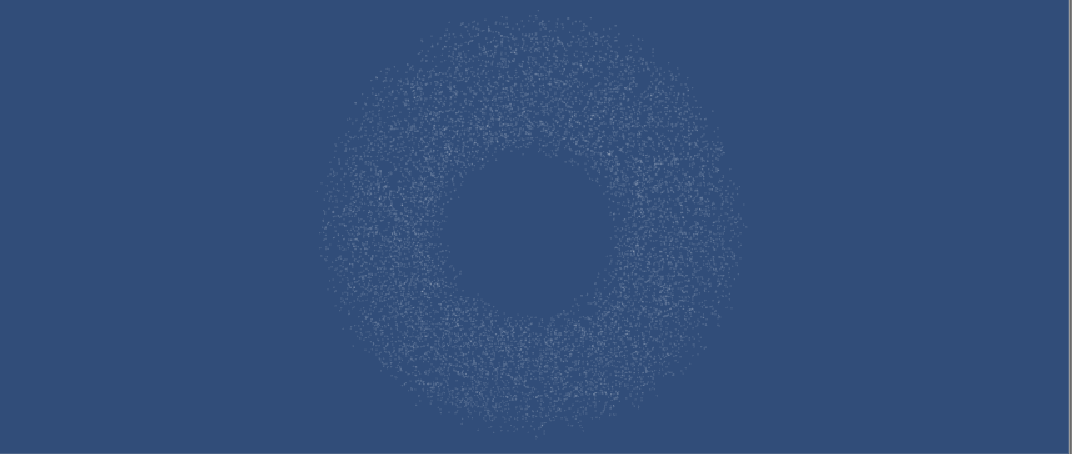# unity3d粒子系统水流

2017-12-16 17:55:22 sinat_20559947 阅读数 10785
• ###### Unity 值得看的500+ 技术内容列表

Unity3D是由Unity Technologies开发的一个让玩家轻松创建诸如三维视频游戏、建筑可视化、实时三维动画等类型互动内容的多平台的综合型游戏开发工具，是一个全面整合的专业游戏引擎。

```Shader "Custom/Stream" {
Properties {
_MainTex ("Albedo (RGB)", 2D) = "white" {}
_MainTint ("Diffuse Tint", Color) = (1,1,1,1)
_ScrollXSpeed ("X Scroll Speed", Range(0,10)) = 2
_ScrollYSpeed ("Y Scroll Speed", Range(0,10)) = 2
}
Tags { "RenderType"="Opaque" }
LOD 200

CGPROGRAM
// Physically based Standard lighting model, and enable shadows on all light types
// Use shader model 3.0 target, to get nicer looking lighting
#pragma target 3.0

// 将类型转换到CG中的可用类型
sampler2D _MainTex;
fixed4 _MainTint;
fixed _ScrollXSpeed;
fixed _ScrollYSpeed;

// 读取模型数据的结构体，名字有些迷惑人
struct Input {
float2 uv_MainTex;
};

// inout类型是一个输出数据的类型，顶点着色器处理好数据之后传递给片段着色器
// 就是通过inout修饰的参数来传递的，也就是说inout修饰的类型是提供给片段着色器进一步渲染的
void surf (Input IN, inout SurfaceOutputStandard o) {

// 先将UV坐标保存起来（这个值的类型是float2或者fixed2都可以）
float2 scrolledUV = IN.uv_MainTex;

// 可以根据自己的需求来决定滚动方向，此处为xy两个方向偏移
// _Time：是基于Unity的系统内置的时间变量
fixed xScrollValue = _ScrollXSpeed * _Time;
fixed yScrollValue = _ScrollYSpeed * _Time;

// fixed2（x，y）：这个就不用过多解释了，将xy合并成一个二元的数据类型
scrolledUV += fixed2(xScrollValue,yScrollValue);

// tex2D（sampler2D，fixed2）官方定义叫：二维纹理查询
// 给出一张图和纹理坐标计算出颜色
half4 c = tex2D (_MainTex, scrolledUV)* _MainTint;
o.Albedo = c.rgb;
o.Alpha = c.a;
}
ENDCG
}
FallBack "Diffuse"
}
```

unity3d粒子系统水流 相关内容

## 【Visual Effect Graph】Unity 2018.3新版粒子系统配置与导入

2019-03-19 09:29:54 u011643463 阅读数 1646
• ###### Unity 值得看的500+ 技术内容列表

Unity3D是由Unity Technologies开发的一个让玩家轻松创建诸如三维视频游戏、建筑可视化、实时三维动画等类型互动内容的多平台的综合型游戏开发工具，是一个全面整合的专业游戏引擎。

unity3d粒子系统水流 相关内容

## Unity3D_新版粒子系统

2018-03-08 14:41:10 bobo553443 阅读数 272
• ###### Unity 值得看的500+ 技术内容列表

Unity3D是由Unity Technologies开发的一个让玩家轻松创建诸如三维视频游戏、建筑可视化、实时三维动画等类型互动内容的多平台的综合型游戏开发工具，是一个全面整合的专业游戏引擎。

## 什么是粒子系统## 新版粒子系统介绍

Shuriken粒子系统是继Unity3.5版本之后推出的新版粒子系统，它采用了模块化管理，个性化的粒子模块配合粒子曲线编辑器使用户更容易创作出各种兵分复杂的粒子效果。## 参数讲解## 简单粒子效果的制作

unity3d粒子系统水流 相关内容

## Unity3D课程——粒子系统与流动效果

2019-11-04 09:30:06 diosmai_kingso 阅读数 586
• ###### Unity 值得看的500+ 技术内容列表

Unity3D是由Unity Technologies开发的一个让玩家轻松创建诸如三维视频游戏、建筑可视化、实时三维动画等类型互动内容的多平台的综合型游戏开发工具，是一个全面整合的专业游戏引擎。

## 粒子光环制作

### 实现步骤

• 在场景中创建一个空对象，并将其命名为ParticleHalo，再在其下创建一个Particle System子对象，命名为Halo
• 两个新的脚本，分别命名为ParticleData和ParticleHalo

#### ParticleData.cs

``````using System.Collections;
using System.Collections.Generic;
using UnityEngine;

public class ParticleData : MonoBehaviour
{
public float angle = 0f;
public float speed = 0f;
public bool clockwise = false;

public ParticleData(float _angle,float _radius, float _speed, bool _clockwise)
{
angle = _angle;
speed = _speed;
clockwise = _clockwise;
}
}
``````

#### ParticleHalo.cs

• 参数：
``````private ParticleSystem par_sys;
private ParticleSystem.MainModule main;
private ParticleSystem.Particle[] halo;
private ParticleData[] par_data;

public float size = 0.05f;              //粒子大小
public float time = 9999f;				//粒子生命周期
public int par_count = 10000;			//粒子数量
``````
• Start：初始化粒子系统，设置粒子系统参数，并发射粒子
``````void Start()
{
halo = new ParticleSystem.Particle[par_count];
par_data = new ParticleData[par_count];

//初始化粒子系统
par_sys = this.GetComponent<ParticleSystem>();
main = par_sys.main;
main.loop = false;
main.startSpeed = 0f;
main.startSize = size;
main.maxParticles = par_count;
par_sys.Emit(par_count);
par_sys.GetParticles(halo);

randomPlace();
}
``````
• randomPlace：将粒子发射到环的不同位置上
``````//随机放置粒子
private void randomPlace()
{
for(int i = 0; i < par_count; i++)
{
float r = Random.Range(5f, 12f);
if (r % 3 == 1)
r = Random.Range(7f, 10f);
else if(r%3==2)
r = Random.Range(8f, 9f);
float angle = Random.Range(0f, 360f);
float theta = angle / 180 * Mathf.PI;
float speed = Random.Range(0.5f, 1.5f);
int flag = Random.Range(0, 2);
bool clockwise = (flag == 0) ? false : true;

halo[i].position = new Vector3(r * Mathf.Cos(theta), r * Mathf.Sin(theta), 0f);
par_data[i] = new ParticleData(angle, r, speed, clockwise);
}
par_sys.SetParticles(halo, halo.Length);
}
``````
• Update：实现每个粒子的运动，包括运动方向，绕圆心旋转以及游离运动
``````void Update()
{
for (int i = 0; i < par_count; i++)
{
if (par_data[i].clockwise)			//顺时针
par_data[i].angle -= Random.Range(0.01f, 0.5f);
else                                //逆时针
par_data[i].angle += Random.Range(0.01f, 0.5f);
par_data[i].angle %= 360f;
float theta = par_data[i].angle / 180 * Mathf.PI;

//粒子进行游离
r += Mathf.PingPong(Time.time * par_data[i].speed, 2f);

halo[i].position = new Vector3(r * Mathf.Cos(theta), r * Mathf.Sin(theta), 0);
}

par_sys.SetParticles(halo, halo.Length);
}
``````

### 最终结果github传送门：传送门

unity3d粒子系统水流 相关内容

## Unity粒子系统——简易特效制作（三）

2018-08-22 09:57:37 qq_40343451 阅读数 1773
• ###### Unity 值得看的500+ 技术内容列表

Unity3D是由Unity Technologies开发的一个让玩家轻松创建诸如三维视频游戏、建筑可视化、实时三维动画等类型互动内容的多平台的综合型游戏开发工具，是一个全面整合的专业游戏引擎。unity3d粒子系统水流 相关内容